NCERT Solutions for Class 8 Maths Chapter 2 Linear Equations in One Variable Ex 2.6 are part of NCERT Solutions for Class 8 Maths. Here we have given NCERT Solutions for Class 8 Maths Chapter 2 Linear Equations in One Variable Ex 2.6.

 Board CBSE Textbook NCERT Class Class 8 Subject Maths Chapter Chapter 2 Chapter Name Linear Equations in One Variable Exercise Ex 2.6 Number of Questions Solved 3 Category NCERT Solutions

## NCERT Solutions for Class 8 Maths Chapter 2 Linear Equations in One Variable Ex 2.6

Question 1.
Solve the following equations:Solution.
1. $$\frac { 8x-3 }{ 3x } =2$$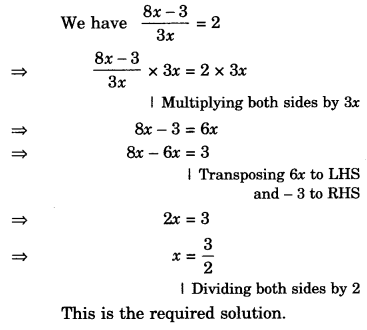2. $$\frac { 9x }{ 7-6x } =15$$3. $$\frac { z }{ z+15 } =\frac { 4 }{ 9 }$$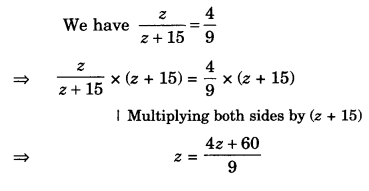4. $$\frac { 3y+4 }{ 2-6y } =\frac { -2 }{ 5 }$$5. $$\frac { 7y+4 }{ y+2 } =\frac { -4 }{ 3 }$$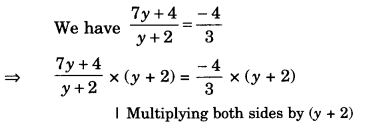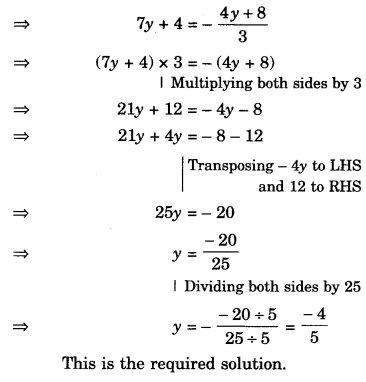Question 2.
The ages of Hari and Harry are in the ratio 5: 7. Four years from now the ratio of their ages will be 3 :4. Find their present ages.
Solution.
Let the present ages of Hari and Harry be 5x years and 7x years respectively.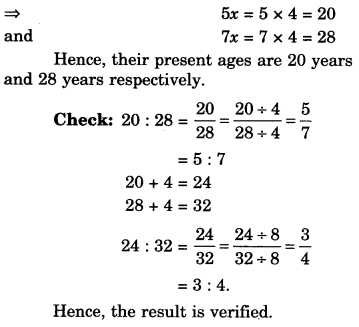Question 3.
The denominator of a rational number is greater than its numerator by 8. If the numerator is increased by 17 and the denominator is decreased by 1, the number obtained is $$\frac { 3 }{ 2 }$$. Find the rational number.
Solution.We hope the NCERT Solutions for Class 8 Maths Chapter 2 Linear Equations in One Variable Ex 2.6 help you. If you have any query regarding NCERT Solutions for Class 8 Maths Chapter 2 Linear Equations in One Variable Ex 2.6, drop a comment below and we will get back to you at the earliest.